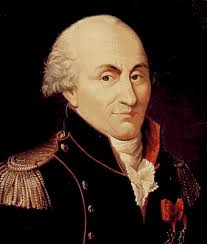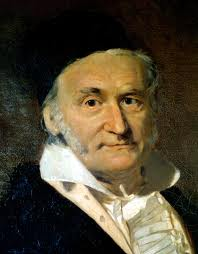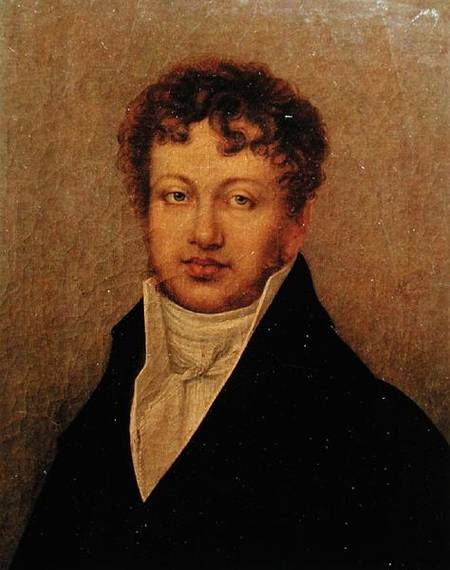PHYS1022 - Electricity and Magnetism Homepage

# PHYS1022 - Electricity and Magnetism - Help with IntegrationThis page provides you with some resources to help with the integration pieces of the course.

## Resources:

• Revision notes on integration here
• A video introducing the idea of using integration to add up
• A video describing two examples of using integration to add up in one dimension
• A video describing how to compute the area of a square using integration
• A video following on from the last one in which the charge of a square is computed assuming some no-uniform charge distribution
• Introduction to 2d polar coordinates and the infinitessimal 2d area element
• Following on from the previous video I compute the area of a circle by integration
• Introduction to 3d polar coordinates
• The 3d infinitessimal volume element and the volume of a sphere

Nick Evans email: evans@soton.ac.uk
Dept. of Physics,
University of Southampton,
Highfield, work tel: (023) 8059 2092
Southampton, SO17 1BJ
U.K. fax: (023) 8059 3910